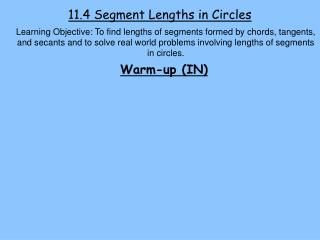DownloadDownload Presentation11.4 Segment Lengths in Circles

# 11.4 Segment Lengths in Circles

Download Presentation## 11.4 Segment Lengths in Circles

- - - - - - - - - - - - - - - - - - - - - - - - - - - E N D - - - - - - - - - - - - - - - - - - - - - - - - - - -
##### Presentation Transcript

1. 11.4 Segment Lengths in Circles Learning Objective: To find lengths of segments formed by chords, tangents, and secants and to solve real world problems involving lengths of segments in circles. Warm-up (IN)

2. Learning Objective: To find lengths of segments formed by chords, tangents, and secants and to solve real world problems involving lengths of segments in circles. . Notes Lengths of chords and secants –

3. Learning Objective: To find lengths of segments formed by chords, tangents, and secants and to solve real world problems involving lengths of segments in circles. Lengths of Tangents and Secants –

4. Learning Objective: To find lengths of segments formed by chords, tangents, and secants and to solve real world problems involving lengths of segments in circles. Ex 1 – Find RT and ST

5. Learning Objective: To find lengths of segments formed by chords, tangents, and secants and to solve real world problems involving lengths of segments in circles. Ex 2 – In the figure below, LN=14.2 and PN=13.5. Find MN and ON.

6. Learning Objective: To find lengths of segments formed by chords, tangents, and secants and to solve real world problems involving lengths of segments in circles. Ex 3–When the GPS system was designed, engineers wanted to minimize both the number of satellites and the distance that the transmitters needed to send signals. If a satellite is at an altitude of 9250 miles, how far do the transmitters need to be able to send signals? D A 9250 mi B 8000 mi C P E

7. Out – Describe how to find the length of a tangent segment using the theorems in this section. Summary – Now, I can figure out… HW –